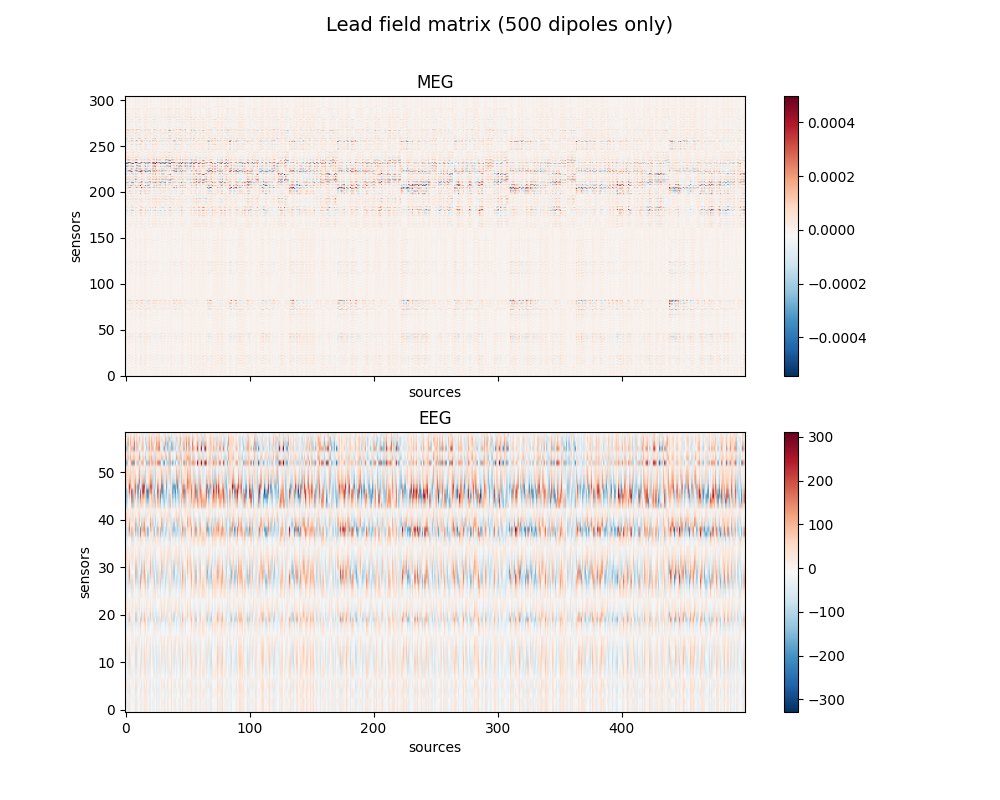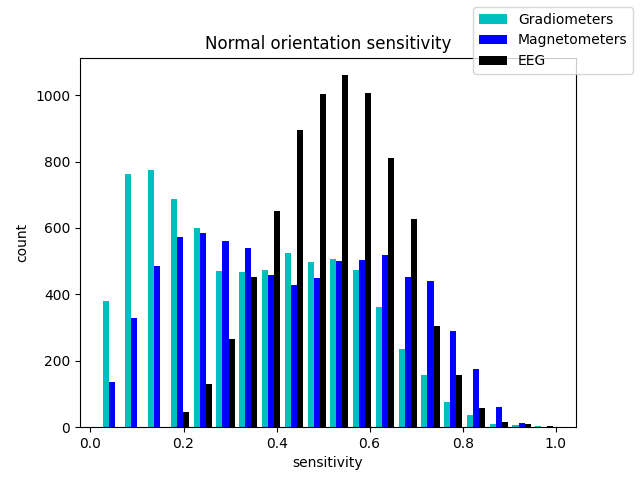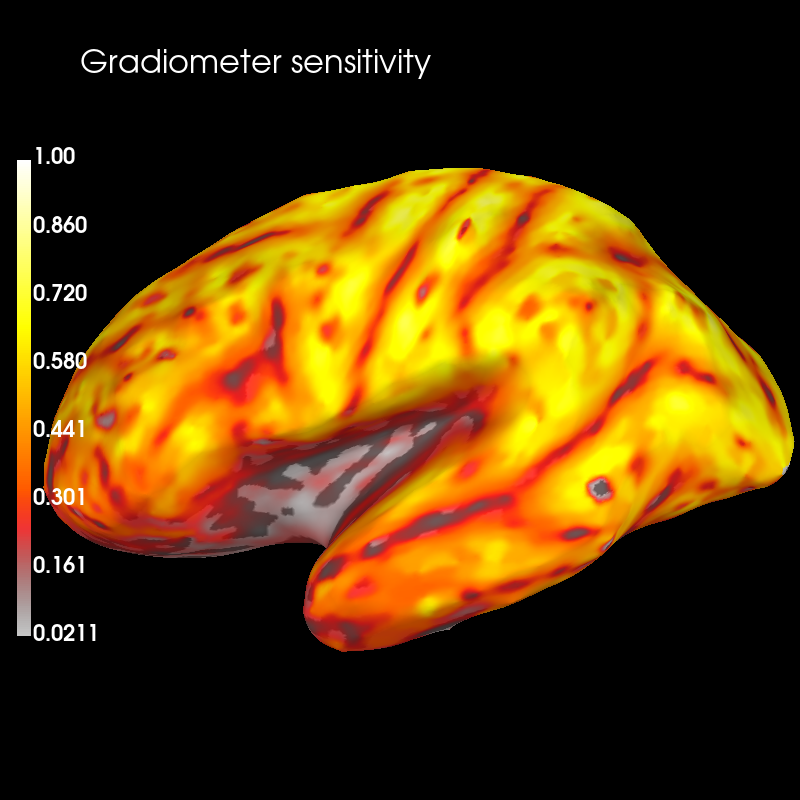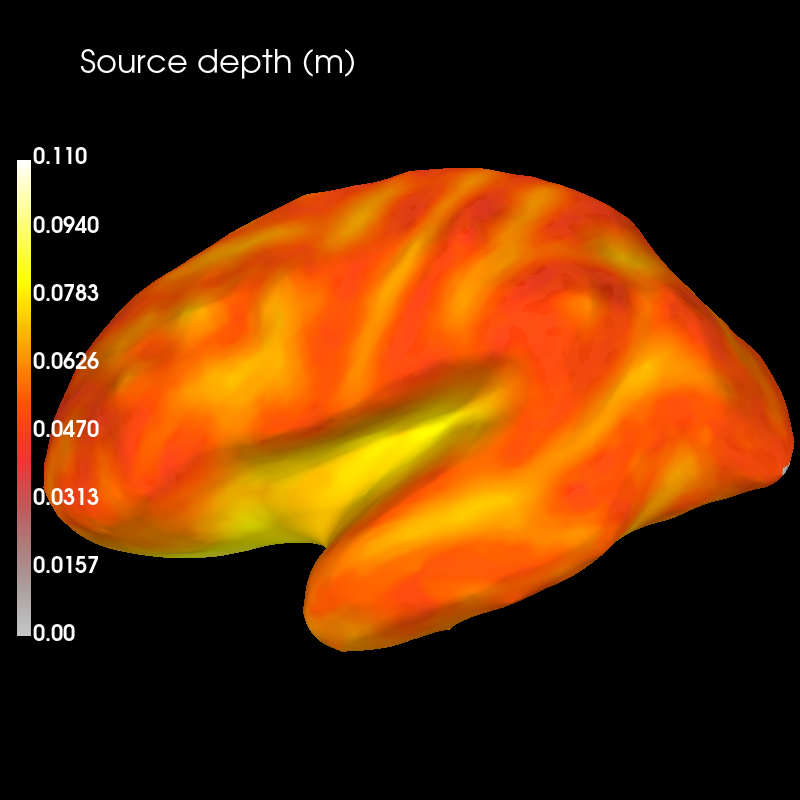# Display sensitivity maps for EEG and MEG sensors#

Sensitivity maps can be produced from forward operators that indicate how well different sensor types will be able to detect neural currents from different regions of the brain.

To get started with forward modeling see Head model and forward computation.

```# Author: Eric Larson <larson.eric.d@gmail.com>
#
```
```import numpy as np
import mne
from mne.datasets import sample
from mne.source_space import compute_distance_to_sensors
from mne.source_estimate import SourceEstimate
import matplotlib.pyplot as plt

print(__doc__)

data_path = sample.data_path()
meg_path = data_path / "MEG" / "sample"
fwd_fname = meg_path / "sample_audvis-meg-eeg-oct-6-fwd.fif"
subjects_dir = data_path / "subjects"

# Read the forward solutions with surface orientation
mne.convert_forward_solution(fwd, surf_ori=True, copy=False)
```
```Reading forward solution from /home/circleci/mne_data/MNE-sample-data/MEG/sample/sample_audvis-meg-eeg-oct-6-fwd.fif...
Computing patch statistics...
[done]
Computing patch statistics...
[done]
Desired named matrix (kind = 3523) not available
Read MEG forward solution (7498 sources, 306 channels, free orientations)
Desired named matrix (kind = 3523) not available
Read EEG forward solution (7498 sources, 60 channels, free orientations)
Forward solutions combined: MEG, EEG
Source spaces transformed to the forward solution coordinate frame
Average patch normals will be employed in the rotation to the local surface coordinates....
Converting to surface-based source orientations...
[done]
Leadfield size : 366 x 22494
```

Compute sensitivity maps

```grad_map = mne.sensitivity_map(fwd, ch_type="grad", mode="fixed")
mag_map = mne.sensitivity_map(fwd, ch_type="mag", mode="fixed")
eeg_map = mne.sensitivity_map(fwd, ch_type="eeg", mode="fixed")
```
```    204 out of 366 channels remain after picking
102 out of 366 channels remain after picking
60 out of 366 channels remain after picking
```

Show gain matrix a.k.a. leadfield matrix with sensitivity map

```picks_meg = mne.pick_types(fwd["info"], meg=True, eeg=False)
picks_eeg = mne.pick_types(fwd["info"], meg=False, eeg=True)

fig, axes = plt.subplots(2, 1, figsize=(10, 8), sharex=True)
fig.suptitle("Lead field matrix (500 dipoles only)", fontsize=14)
for ax, picks, ch_type in zip(axes, [picks_meg, picks_eeg], ["meg", "eeg"]):
im = ax.imshow(leadfield[picks, :500], origin="lower", aspect="auto", cmap="RdBu_r")
ax.set_title(ch_type.upper())
ax.set_xlabel("sources")
ax.set_ylabel("sensors")
fig.colorbar(im, ax=ax)

fig_2, ax = plt.subplots()
ax.hist(
bins=20,
color=["c", "b", "k"],
)
fig_2.legend()
ax.set(title="Normal orientation sensitivity", xlabel="sensitivity", ylabel="count")

subjects_dir=subjects_dir, clim=dict(lims=[0, 50, 100]), figure=1
)
```
••```Using control points [0.02108201 0.32186553 1.        ]
```

Compare sensitivity map with distribution of source depths

```# source space with vertices
src = fwd["src"]

# Compute minimum Euclidean distances between vertices and MEG sensors
depths = compute_distance_to_sensors(src=src, info=fwd["info"], picks=picks_meg).min(
axis=1
)
maxdep = depths.max()  # for scaling

vertices = [src["vertno"], src["vertno"]]

depths_map = SourceEstimate(data=depths, vertices=vertices, tmin=0.0, tstep=1.0)

brain_dep = depths_map.plot(
subject="sample",
subjects_dir=subjects_dir,
clim=dict(kind="value", lims=[0, maxdep / 2.0, maxdep]),
figure=2,
)
brain_dep.add_text(0.1, 0.9, "Source depth (m)", "title", font_size=16)
```Sensitivity is likely to co-vary with the distance between sources to sensors. To determine the strength of this relationship, we can compute the correlation between source depth and sensitivity values.

```corr = np.corrcoef(depths, grad_map.data[:, 0])[0, 1]
print("Correlation between source depth and gradiomter sensitivity values: %f." % corr)
```
```Correlation between source depth and gradiomter sensitivity values: -0.815476.
```

Gradiometer sensitiviy is highest close to the sensors, and decreases rapidly with inreasing source depth. This is confirmed by the high negative correlation between the two.

Total running time of the script: ( 0 minutes 14.515 seconds)

Estimated memory usage: 135 MB

Gallery generated by Sphinx-Gallery